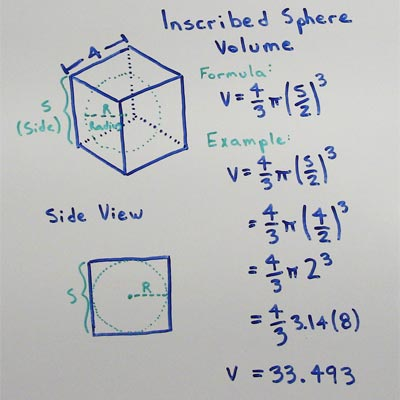# Volume of Cube

## Inscribed Sphere Volume

A cube with a side length (S) of 5 has an inscribed sphere with a volume (V) of 65.4498.

## Inscribed Sphere Volume Formula

the Volume of a sphere with radius (R) can be found with this formula

• V = (4/3)πR3

the radius (R) of a sphere inscribed in a cube with side length S can be found with this formula

• R = S/2

by combining the two formulae we can determine the Volume of an inscribed sphere in terms of cube Side length

• V = (4/3)π(S/2)3Given a cube with a side length S the volume (V) of an inscribed sphere can be found by substituting the formula for finding the Radius of an inscribed sphere into the formula for finding the Volume of a sphere.

### Formulas

What is the formula for the volume of a Cube?

V = S3

What is the formula for the volume of a Rectangular Prism?

V = L x W x H

### Other Formulae

for cubes with side length S

Cube Surface Area

6S2
Cube Face Diagonal
(√2)S
Cube Space Diagonal
(√3)S
S/2
All formulae...